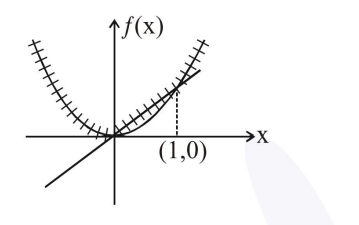# Solve this following

Question:

Let $f: \mathrm{R} \rightarrow \mathrm{R}$ be a function defined by $f(x)=\max \left\{x, x^{2}\right\}$. Let $S$ denote the set of all points in R, where $f$ is not differentiable. Then :

1. $\{0,1\}$

2. $\{0\}$

3. $\phi($ an empty set $)$

4. $\{1\}$

Correct Option: 1

Solution:

$f(x)=\max \left(x, x^{2}\right)$Non-differentiable at $x=0,1$

$\mathrm{S}=\{0,1\}$